lvy>試卷(2022/08/12)

# 104 年 - 104 國立中山大學_碩士班招生考試_電機系(丁組)：電力工程#110208

【非選題】
1.

1. The impedance matrix (ZBus) for Fig.I can be expressed as eq. (1). (25%)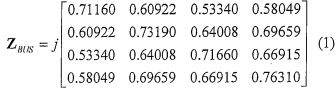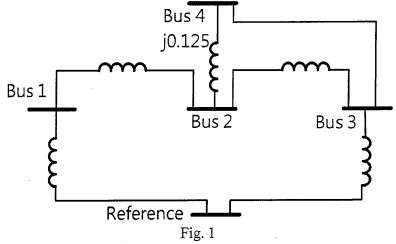【題組】 (a) Calculate the Z matrix after the line between Bus 2 and Bus 4 is removed； (15%)

【非選題】
2.【題組】(b) Assuming the bus voltages before fault are all 1.0p.u. Calculate the short circuit current when a three-phase short-circuit fault occurred at Bus 3 for (a) and bus voltages after this fault. (10%)

【非選題】
3.

2.A Y-connected load bank with a three-phase rating of 1000 kVA and 1150 V consists of three identical resistors of 1.3225 ohms. The load bank has the following applied voltages: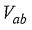=920∠2.8° ,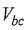=1380∠-41.4° , and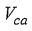=11502180 V. Determine (15%)

【題組】

(a) the zero-, positive- and negative-sequence line-to-neutral voltages； (5%)

【非選題】
4.【題組】

(b) the zero-, positive- and negative-sequence line-to-line voltages； (5%)

【非選題】
5.【題組】

(c) the zero-, positive- and negative-sequence line currents. (5%) (All answers should be in p.u.)

【非選題】
6.

3.Calculate the line flows and power flows from Bus i to Bus j and Bus j to Bus i for Fig. 2 and the line loss for this line (all values in Fig. 2 are in p.u.). (20%)【非選題】
7.

4. The inertia constant (H) of a three-phase 60-Hz generator is 1.5 p.u.-s. The electrical power delivery by the generator (Pe) versus its power angle (δ ) can be expressed as eq. (2). The mechanical power input to the generator ( Pm) is 0.5 p.u. (25%)

Pe=1.2319sin(δ)

【題組】 (a) Determine the swing equation and the initial operating power angle. (10%)

【非選題】
8.【題組】(b) Calculate the critical clearing time for the generator when a three-phase bolted short-circuit at the generator terminals causes Pe =0. (15%)

【非選題】
9.

5. Draw the phasor diagrams between the voltages and current of a synchronous generator of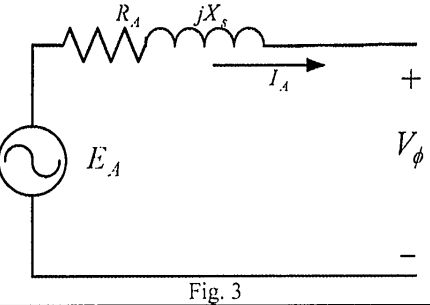【題組】 (a) unity power factor,

【非選題】
10.【題組】(b) lagging power factor and

【非選題】
11.【題組】(c) leading power factor. (15%)

### 懸賞詳解

#### 國一自然上第三次

25. 日正當中的沙漠，蜥蜴躲在石頭下方的陰影下，企圖吐出舌頭捕捉食物―螞蟻，但蜥蜴不肯離開陰影，所以捕 捉不成，只能眼巴巴看著螞蟻逃跑。�...